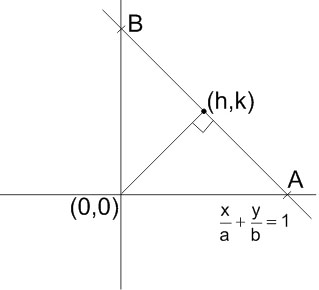# Let a and b be any two numbers satisfyingThen,   the   foot   of perpendicular from the origin on the variable line,, lies on : Option 1) a hyperbola with each semi-axis =Option 2)  a hyperbola with each semi-axis = 2. Option 3)     a circle of radius = 2 Option 4)    a circle of radius =As we learnt in

Equation of a line perpendicular to a given line -is the line perpendicular to.

- whereinis some other constant than.Equation of line perpendicular to ABax - by = 0

Foot of perpendicular isand ax - by = 0Also,Option 1)

a hyperbola with each semi-axis =This is incorrect option

Option 2)

a hyperbola with each semi-axis = 2.

This is incorrect option

Option 3)

a circle of radius = 2

This is correct option

Option 4)

a circle of radius =This is incorrect option

### Preparation Products

##### JEE Main Rank Booster 2021

This course will help student to be better prepared and study in the right direction for JEE Main..

₹ 13999/- ₹ 9999/-
##### Knockout JEE Main April 2021 (Easy Installments)

An exhaustive E-learning program for the complete preparation of JEE Main..

₹ 4999/-
##### Knockout JEE Main April 2021

An exhaustive E-learning program for the complete preparation of JEE Main..

₹ 22999/- ₹ 14999/-
##### Knockout JEE Main April 2022

An exhaustive E-learning program for the complete preparation of JEE Main..

₹ 34999/- ₹ 24999/-